# Insertion at end of circular linked list

Problem Statement: Given a Circular Linked List, insert an element at end of the circular linked list.

Examples:

```Example 1:
Input: 1 2 3 4 5 6, newnode=11
Output: 1 2 3 4 5 6 11

Example 2:
Input: NULL, newnode=10
Output: 10```

### Solution:

DisclaimerDon’t jump directly to the solution, try it out yourself first.

### Solution 1:

Approach: O(n) approach

In this approach, we’ll iterate to the last node and perform the following operations:

1. Make the next pointer of the last node to point to the newnode.
2. Make the next pointer of the newnode to point to the head node.
3. Return the head of the linked list.

The following diagram gives a better understanding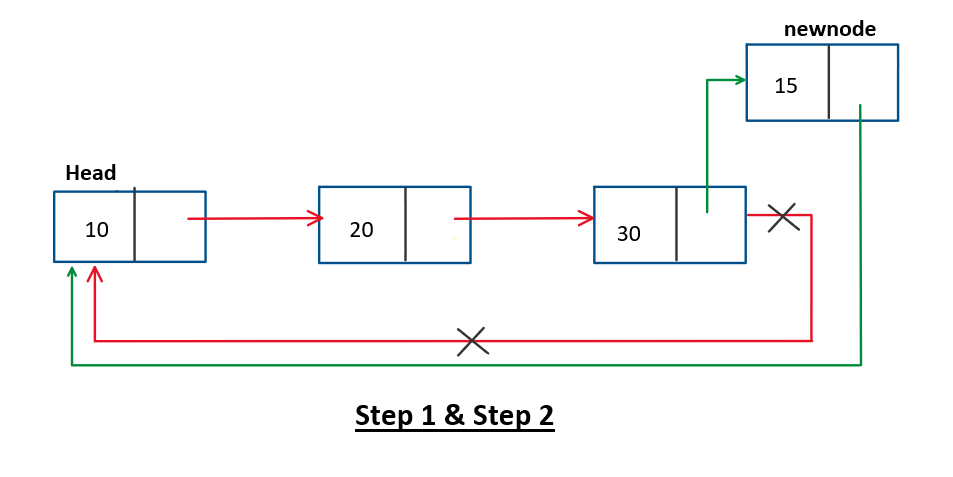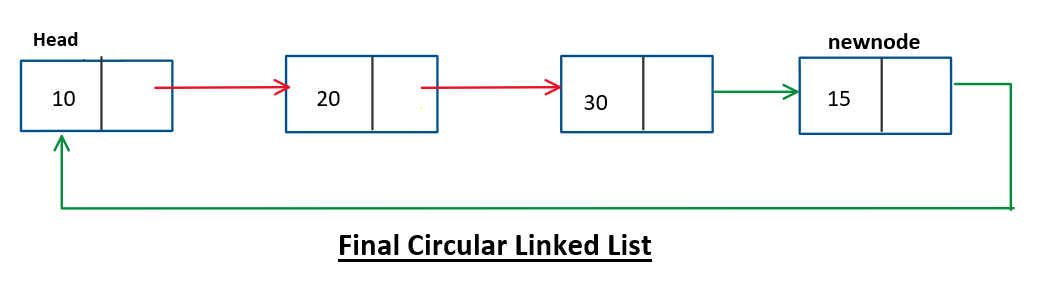Code:

## C++ Code

``````#include<iostream>
using namespace std;

struct node {
int data;
node * next;
node(int d) {
data = d;
next = NULL;
}
};
void print(node * head) {
if (head == NULL) return;
cout << head -> data << " ";
for (node * current = head -> next; current != head; current = current -> next) {
cout << current -> data << " ";
}
cout<<endl;
}
// Approach 1: O(n)
node * insertion_end(node * head, int x) {
node * newnode = new node(x);
if (head == NULL) {
newnode -> next = newnode;
return newnode;
} else {
node * current = head;
while (current -> next != head) {
current = current -> next;
}
current -> next = newnode;
newnode -> next = head;
}
}
int main() {
node * head = new node(10);
head -> next = new node(20);
head -> next -> next = new node(30);
head -> next -> next -> next = head;
cout<<"Before insertion:"<<endl;
cout<<"After insertion:"<<endl;
return 0;
}
``````

Output:

Before insertion:
10 20 30
After insertion:
10 20 30 40

Time Complexity: O(n)

Space Complexity: O(1)

## Java Code

``````class node {
int data;
node next;
node(int d) {
data = d;
next = null;
}
}
public class Main {
public static void main(String args[]) {
node head = new node(10);
head.next = new node(20);
head.next.next = new node(30);
System.out.println("Before insertion: ");
System.out.println("After insertion: ");

}
static node insertion_end(node head, int x) {
node newnode = new node(x);
if (head == null) {
newnode.next = newnode;
return newnode;
}
node current = head;
while (current.next != head) {
current = current.next;
}
current.next = newnode;
}
static void printlist(node head) {
node current = head;
do {
System.out.print(current.data + " ");
current = current.next;
} while (current != head);
System.out.println();
}
}
``````

Output:

Before insertion:
10 20 30
After insertion:
10 20 30 40

Time Complexity: O(n)

Space Complexity: O(1)

### Solution 2:

Approach 2: O(1) approach

This approach would be a bit tricky for some learners because while reading the steps of this approach, various conflicts would arise in your minds. I’ll try my best to explain this approach both diagrammatically and theoretically for a better understanding.

The steps involved in this approach are as follows:

1. Make the newnode as the second node of the linked list.
2. Swap the data of the newnode and the head node.
3. Return the newnode as the new head of our circular linked list

The following diagram would help in better understanding of the above approach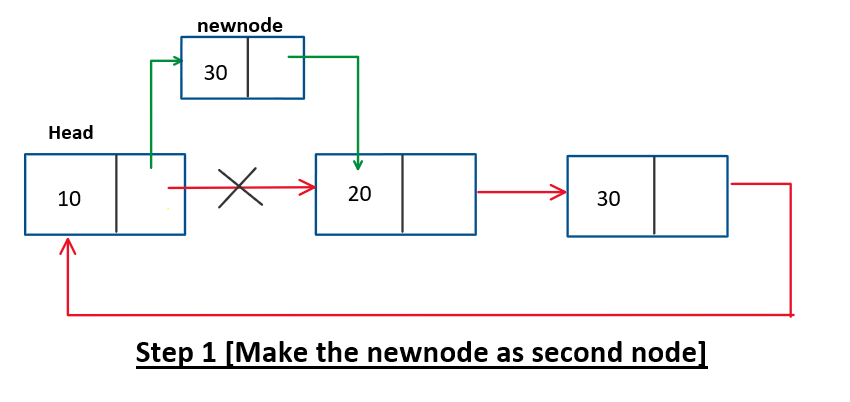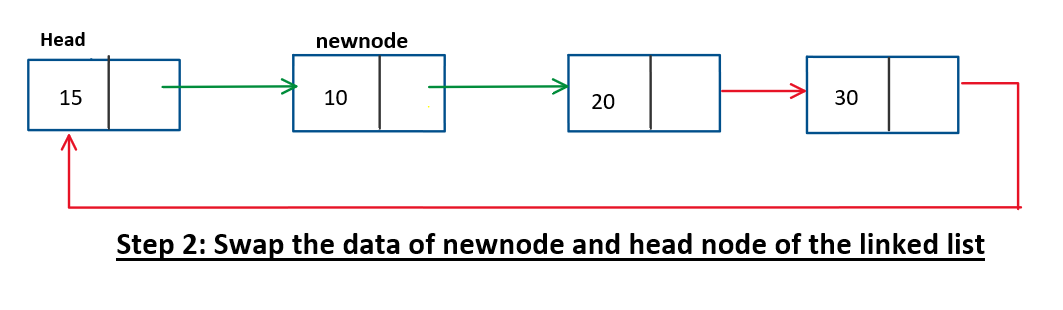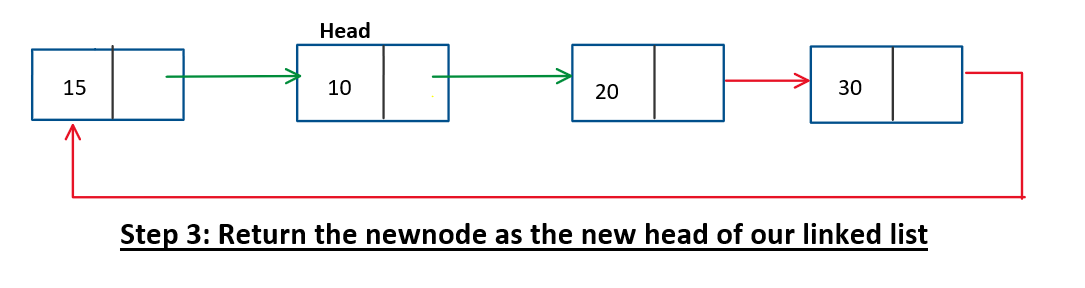If we reconstruct the above diagram, we get something as follows which is our answer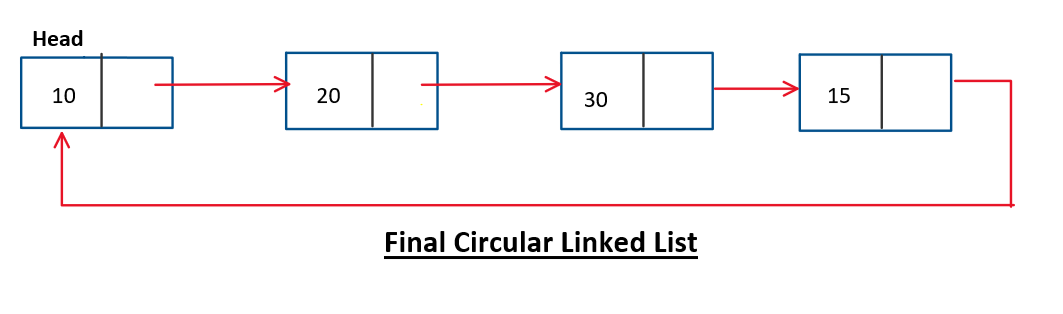There is no difference between the 3rd and 4th diagrams, it only differs in terms of pictorial representation.

Code:

## C++ Code

``````#include<iostream>
using namespace std;

struct node {
int data;
node * next;
node(int d) {
data = d;
next = NULL;
}
};
void print(node * head) {
if (head == NULL) return;
cout << head -> data << " ";
for (node * current = head -> next; current != head; current = current -> next)
{
cout << current -> data << " ";
}
cout<<endl;
}
node * insertion_END(node * head, int x) {
node * newnode = new node(x);
if (head == NULL) {
newnode -> next = newnode;
return newnode;
} else {
// step 1
newnode -> next = head -> next;
head -> next = newnode;

// step 2
int swapper;
swapper = head -> data;
head -> data = newnode -> data;
newnode -> data = swapper;

// step 3
return newnode;
}
}
int main() {
node * head = new node(10);
head -> next = new node(20);
head -> next -> next = new node(30);
head -> next -> next -> next = head;
cout<<"Before insertion:"<<endl;
cout<<"After insertion:"<<endl;
return 0;
}
``````

Output:

Before insertion:
10 20 30
After insertion:
10 20 30 40

Time Complexity: O(1)

Space Complexity: O(1)

## Java Code

``````class node {
int data;
node next;
node(int d) {
data = d;
next = null;
}
}
public class Main {
public static void main(String args[]) {
node head = new node(10);
head.next = new node(20);
head.next.next = new node(30);
System.out.println("Before insertion:");
System.out.println("After insertion:");
}
static node insertion_END(node head, int x) {
node newnode = new node(x);
if (head == null) {
newnode.next = newnode;
return newnode;
}
// step 1

// step 2
int swapper = head.data;
newnode.data = swapper;

// step 3
return newnode;
}
static void printlist(node head) {
node current = head;
do {
System.out.print(current.data + " ");
current = current.next;
} while (current != head);
System.out.println();
}
}
``````

Output:

Before insertion:
10 20 30
After insertion:
10 20 30 40

Time Complexity: O(1)

Space Complexity: O(1)

Special thanks to Yash Mishra for contributing to this article on takeUforward. If you also wish to share your knowledge with the takeUforward fam, please check out this article